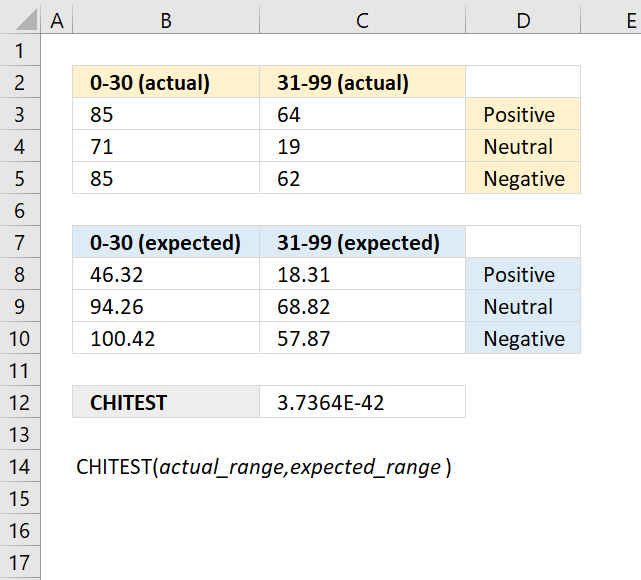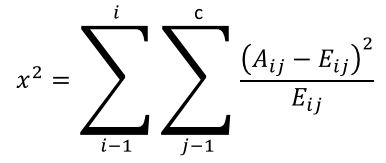Author: Oscar Cronquist Article last updated on May 11, 2022The CHITEST function calculates the test for independence, the value returned from the chi-squared statistical distribution and the correct degrees of freedom. Use this function to check if hypothesized results are valid.

The CHITEST function is outdated and you will find it in the compatibility category, the function has been replaced with the CHISQ.TEST function.

Formula in cell C12:

=CHITEST(B3:C5,B8:C10)

### Excel Function Syntax

CHITEST(actual_range,expected_range)

### Arguments

 actual_range Required. A range of data. expected_range Required. A range of data.

Independence is indicated by a low number of χ2.

The CHITEST function returns

• #N/A error value if the number of data points in the arguments doesn't match.

CHITEST function equation:Aij = actual frequency in the i-th row, j-th column

Eij = expected frequency in the i-th row, j-th column

r = number of rows

c = number of columns